### Home > MC2 > Chapter 3 > Lesson 3.1.1 > Problem3-9

3-9.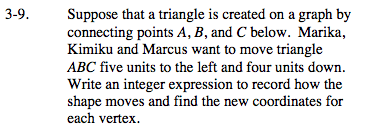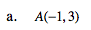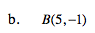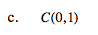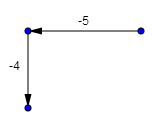Since each point is translated to the left and then down, there is a negative x-coordinate movement and a negative y-coordinate movement.

Think of how you could express the x and y coordinate translation. Would you add or subtract from the original x and y coordinates to get the new translated point?

(a) x: −1+(−5) = −6
y: 3+(−4) = −1